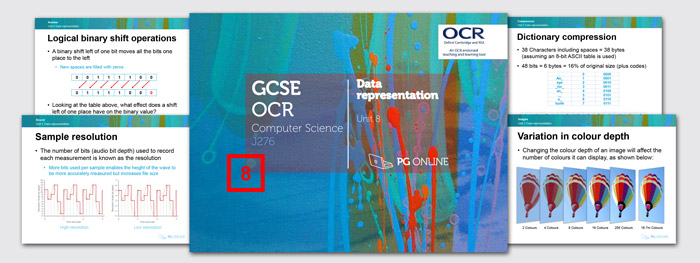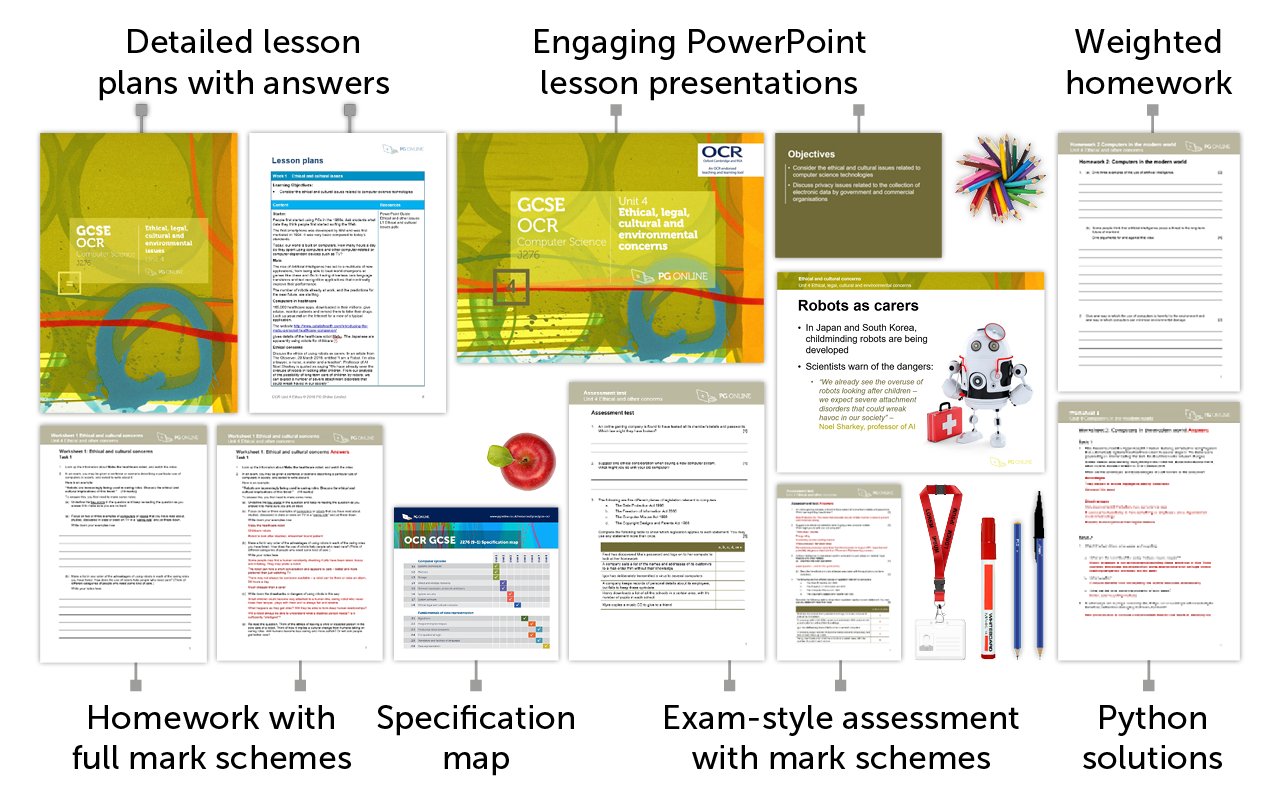GCSE OCR

£120.00 + VAT+

8

Inside the Unit...Areas Covered

• Lesson 1 Storage units and binary numbers
• Lesson 2 Binary arithmetic and hexadecimal
• Lesson 3 ASCII and Unicode
• Lesson 4 Images
• Lesson 5 Sound
• Lesson 6 Compression
• Lesson 7 Assessment

There are 6 worksheets, 6 homework tasks, and an assessment test, each with answers included in this unit.

Summary

The unit is subdivided into six learning hours spread across six lessons, plus a test, in order to fit with most school timetables. It is a theoretical unit covering Section 2.6 of the latest OCR GCSE Computer Science specification. Each lesson contains a worksheet to be done in class to consolidate students’ knowledge and understanding, as well as a homework sheet to give them plenty of practice in answering exam-type questions. The conversion of integers from denary to binary is covered in the first lessons, together with simple binary addition, overflow and shifts. Check digits are also covered by practical example. In subsequent lessons, the use of hexadecimal numbers and the binary representation of characters is described. Representation of images and sound, and compression techniques are covered in three separate lessons. In the final lesson students sit an assessment test comprising questions similar to those found on the OCR exam paper.

What's included in the toolkit?The GCSE units have been written to satisfy the specification for the theoretical Component 2 Computational thinking, algorithms and programming of the new OCR J276 Computing GCSE. Each units contains:

• PowerPoint slides for each lesson
• Detailed lesson plans
• Learning objectives and outcomes
• Worksheets and homework activities with answers
• End-of-unit Assessment test with answers
• Other material and links to online resources

What people say...

Professional looking resources that would enable consistency within the department and the use of worksheet and homework task that comprehensively covered the spec points

Helen Webb. Science teacher, Lutterworth College

The units we have ordered are proving really useful and helpful with development of materials for new specs.  The Maths for D&T have been particularly helpful.

Linda Hill. Design and Technology Teacher, Coundon Court

Inside the Unit...

Areas Covered

• Lesson 1 Storage units and binary numbers
• Lesson 2 Binary arithmetic and hexadecimal
• Lesson 3 ASCII and Unicode
• Lesson 4 Images
• Lesson 5 Sound
• Lesson 6 Compression
• Lesson 7 Assessment

There are 6 worksheets, 6 homework tasks, and an assessment test, each with answers included in this unit.

• How to order

1. Add individual units to a draft order or download a blank order form below to complete manually

2. Using a draft order you can either:

2. Email us your complete order
3. Create a PDF
(to fax or email at a later date)

• Pricing and Discounts

Each unit is individually priced for LIFE. Please view each unit for detail.

Discounts are cumulative based on the total of units licenced to your department.

All prices subject to further discounts and VAT.

There are no annual renewal fees.

 2-5 units 10% discount 6-11 units 15% discount 12+ units 20% discount